Скачать презентацию Physics-Unit 4 -Motion in Two Dimensions Projectile

b997764c1b859c03c7240e000b36e32f.ppt

• Количество слайдов: 37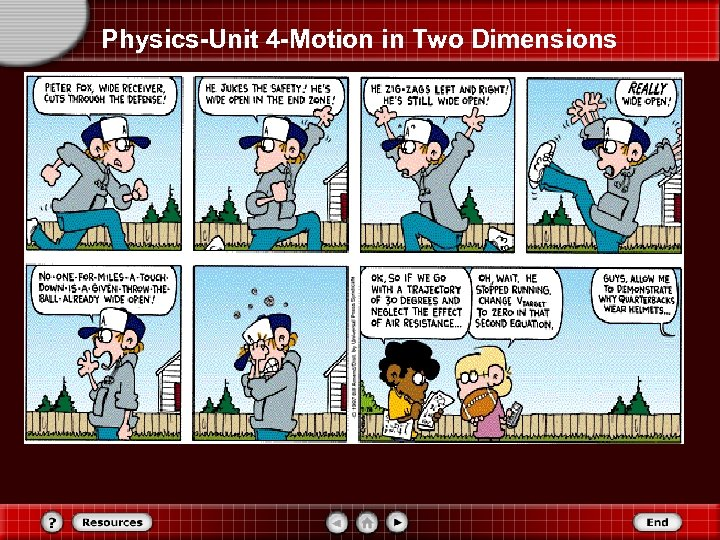Physics-Unit 4 -Motion in Two Dimensions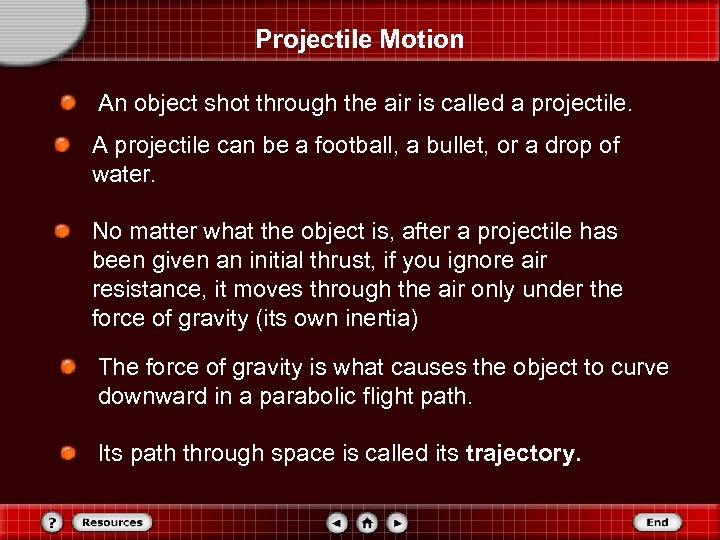Projectile Motion An object shot through the air is called a projectile. A projectile can be a football, a bullet, or a drop of water. No matter what the object is, after a projectile has been given an initial thrust, if you ignore air resistance, it moves through the air only under the force of gravity (its own inertia) The force of gravity is what causes the object to curve downward in a parabolic flight path. Its path through space is called its trajectory.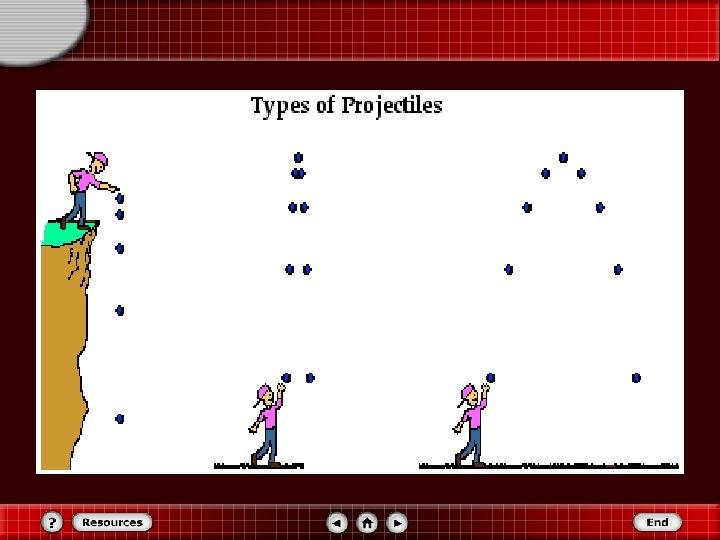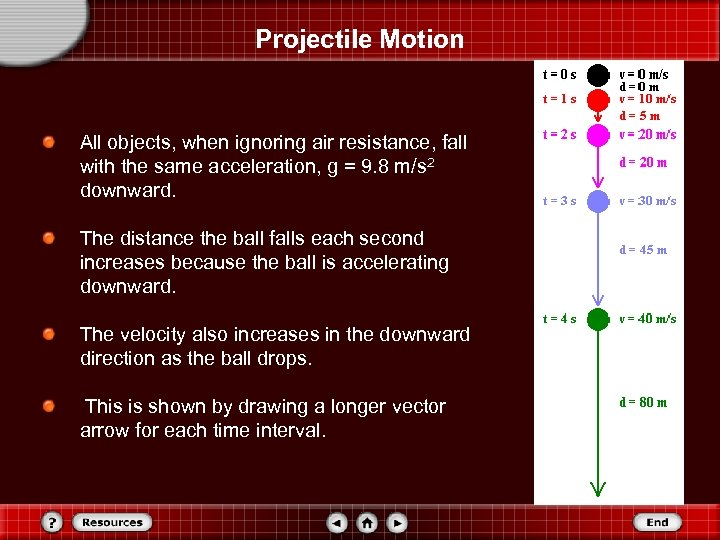Projectile Motion All objects, when ignoring air resistance, fall with the same acceleration, g = 9. 8 m/s 2 downward. The distance the ball falls each second increases because the ball is accelerating downward. The velocity also increases in the downward direction as the ball drops. This is shown by drawing a longer vector arrow for each time interval.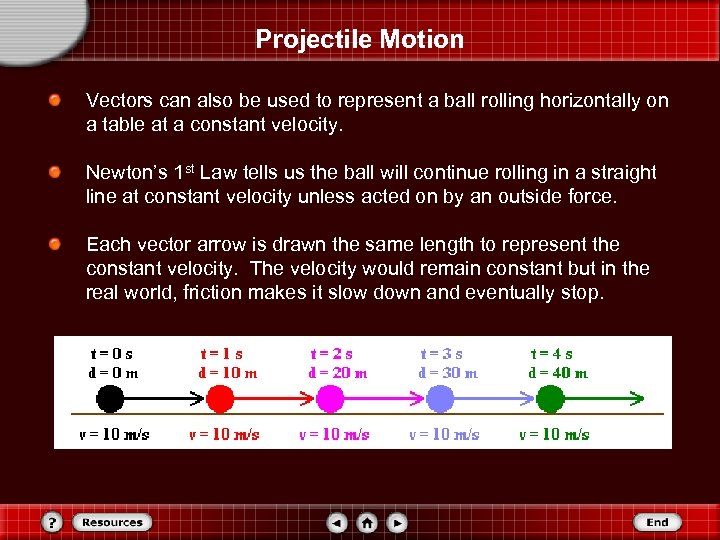Projectile Motion Vectors can also be used to represent a ball rolling horizontally on a table at a constant velocity. Newton’s 1 st Law tells us the ball will continue rolling in a straight line at constant velocity unless acted on by an outside force. Each vector arrow is drawn the same length to represent the constant velocity. The velocity would remain constant but in the real world, friction makes it slow down and eventually stop.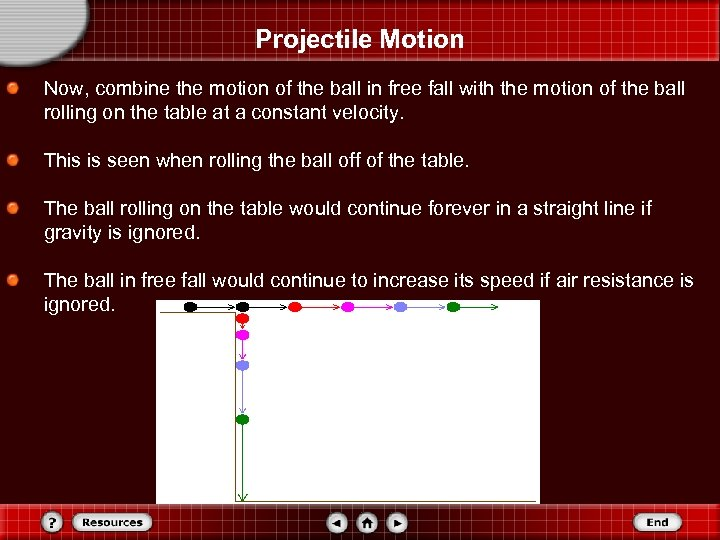Projectile Motion Now, combine the motion of the ball in free fall with the motion of the ball rolling on the table at a constant velocity. This is seen when rolling the ball off of the table. The ball rolling on the table would continue forever in a straight line if gravity is ignored. The ball in free fall would continue to increase its speed if air resistance is ignored.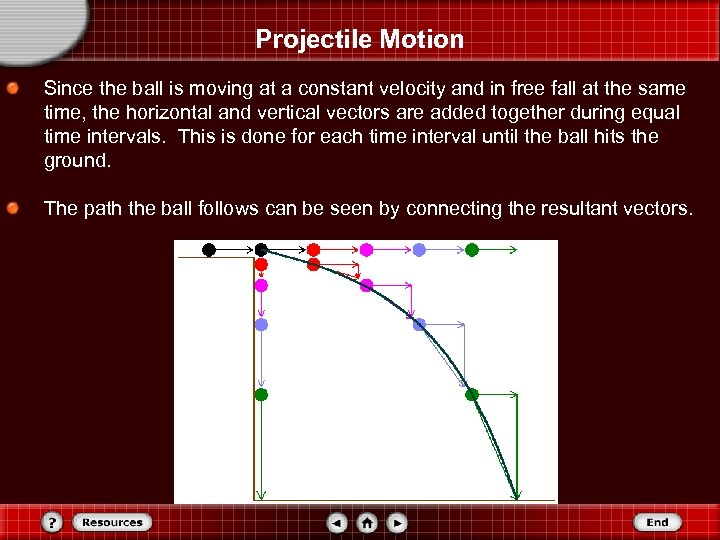Projectile Motion Since the ball is moving at a constant velocity and in free fall at the same time, the horizontal and vertical vectors are added together during equal time intervals. This is done for each time interval until the ball hits the ground. The path the ball follows can be seen by connecting the resultant vectors.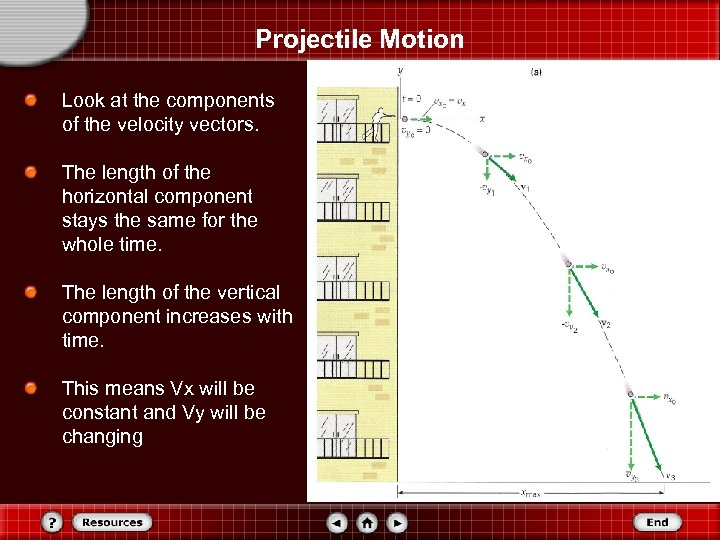Projectile Motion Look at the components of the velocity vectors. The length of the horizontal component stays the same for the whole time. The length of the vertical component increases with time. This means Vx will be constant and Vy will be changing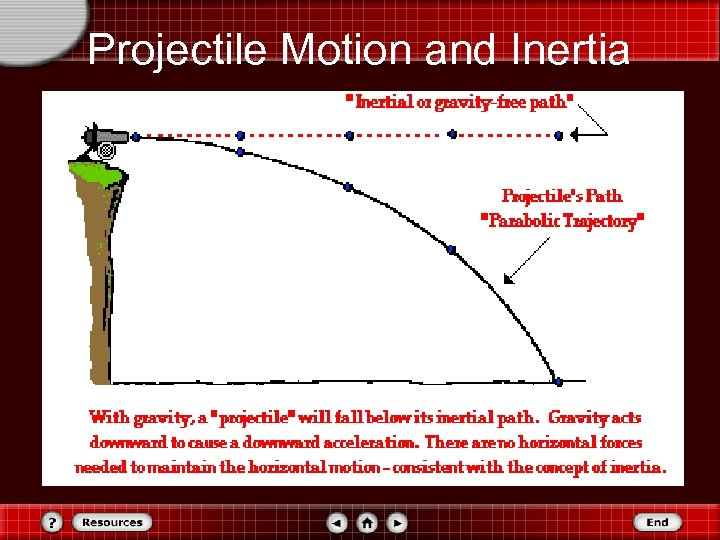Projectile Motion and Inertia •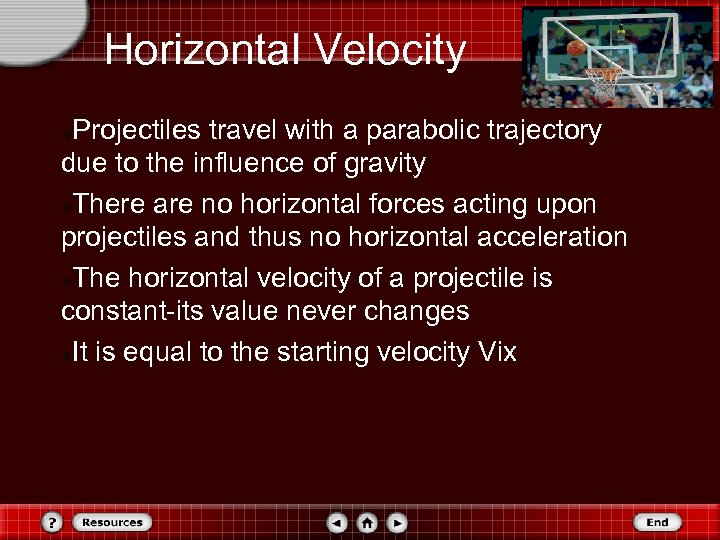Horizontal Velocity Projectiles travel with a parabolic trajectory due to the influence of gravity v. There are no horizontal forces acting upon projectiles and thus no horizontal acceleration v. The horizontal velocity of a projectile is constant-its value never changes v. It is equal to the starting velocity Vix v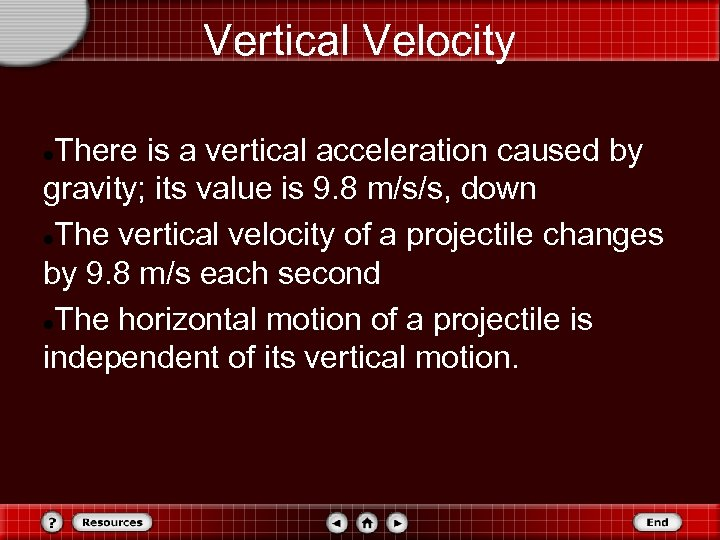Vertical Velocity There is a vertical acceleration caused by gravity; its value is 9. 8 m/s/s, down The vertical velocity of a projectile changes by 9. 8 m/s each second The horizontal motion of a projectile is independent of its vertical motion.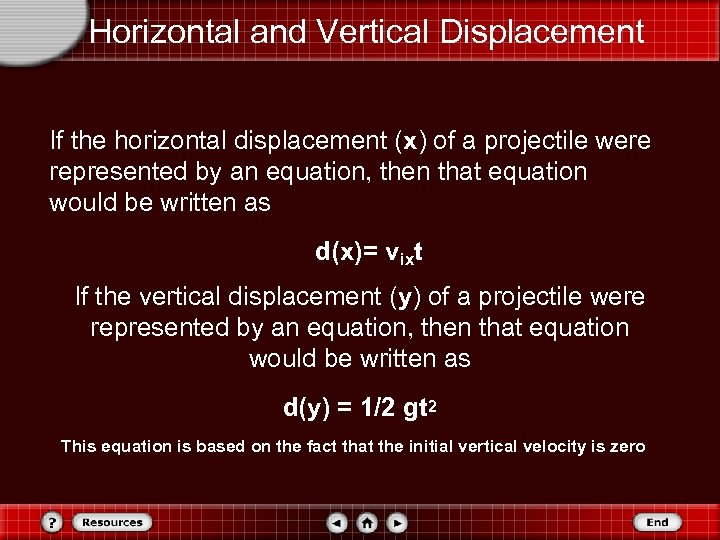Horizontal and Vertical Displacement If the horizontal displacement (x) of a projectile were represented by an equation, then that equation would be written as d(x)= vixt If the vertical displacement (y) of a projectile were represented by an equation, then that equation would be written as d(y) = 1/2 gt 2 This equation is based on the fact that the initial vertical velocity is zero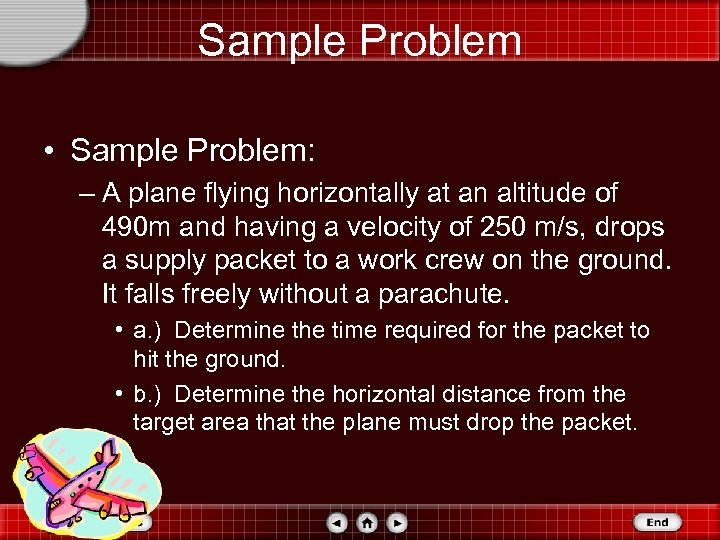Sample Problem • Sample Problem: – A plane flying horizontally at an altitude of 490 m and having a velocity of 250 m/s, drops a supply packet to a work crew on the ground. It falls freely without a parachute. • a. ) Determine the time required for the packet to hit the ground. • b. ) Determine the horizontal distance from the target area that the plane must drop the packet.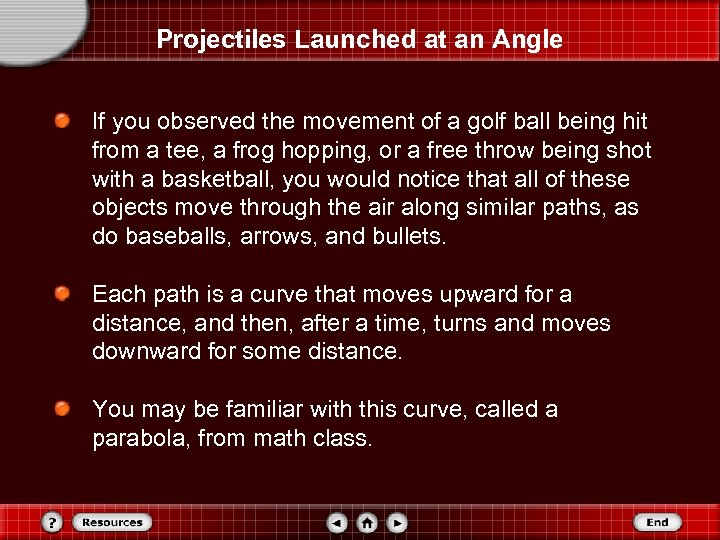Projectiles Launched at an Angle If you observed the movement of a golf ball being hit from a tee, a frog hopping, or a free throw being shot with a basketball, you would notice that all of these objects move through the air along similar paths, as do baseballs, arrows, and bullets. Each path is a curve that moves upward for a distance, and then, after a time, turns and moves downward for some distance. You may be familiar with this curve, called a parabola, from math class.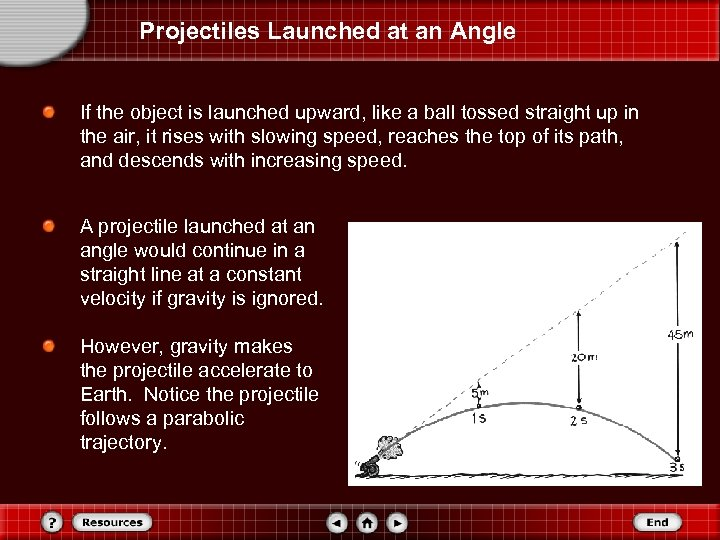Projectiles Launched at an Angle If the object is launched upward, like a ball tossed straight up in the air, it rises with slowing speed, reaches the top of its path, and descends with increasing speed. A projectile launched at an angle would continue in a straight line at a constant velocity if gravity is ignored. However, gravity makes the projectile accelerate to Earth. Notice the projectile follows a parabolic trajectory.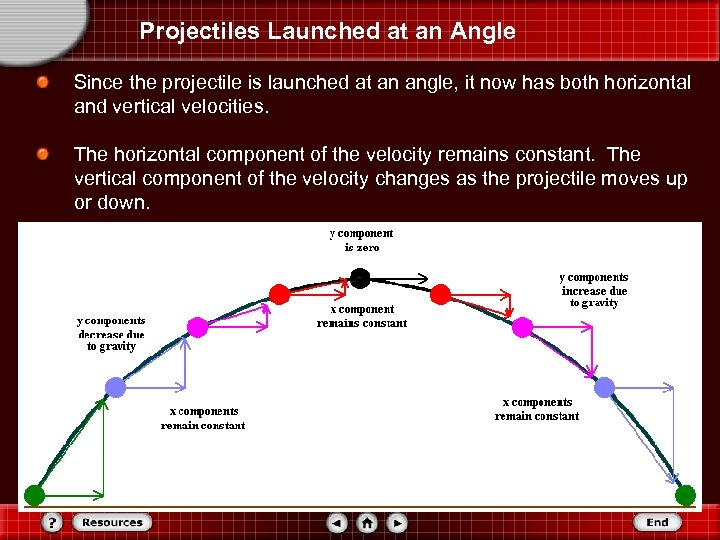Projectiles Launched at an Angle Since the projectile is launched at an angle, it now has both horizontal and vertical velocities. The horizontal component of the velocity remains constant. The vertical component of the velocity changes as the projectile moves up or down.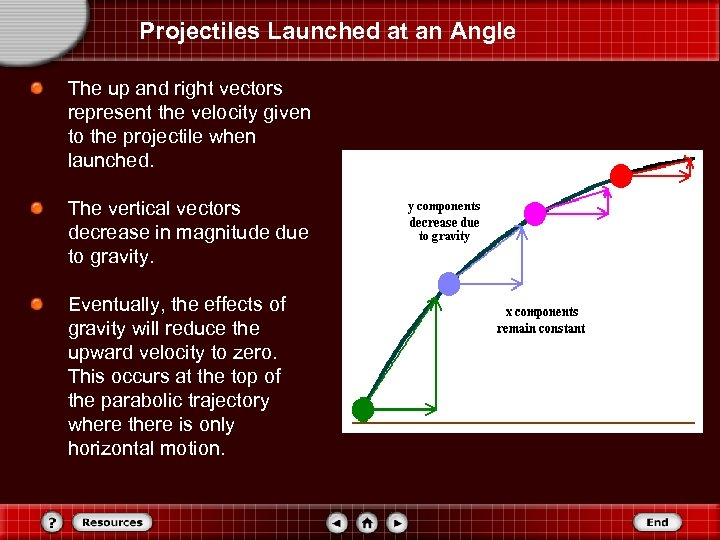Projectiles Launched at an Angle The up and right vectors represent the velocity given to the projectile when launched. The vertical vectors decrease in magnitude due to gravity. Eventually, the effects of gravity will reduce the upward velocity to zero. This occurs at the top of the parabolic trajectory where there is only horizontal motion.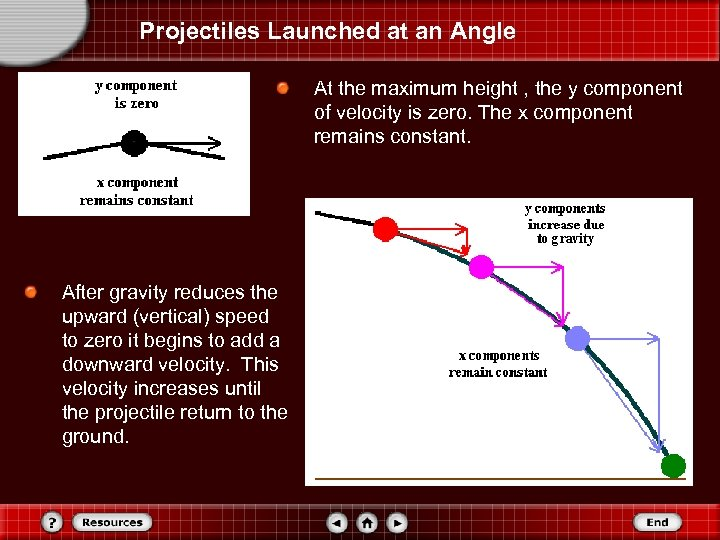Projectiles Launched at an Angle At the maximum height , the y component of velocity is zero. The x component remains constant. After gravity reduces the upward (vertical) speed to zero it begins to add a downward velocity. This velocity increases until the projectile return to the ground.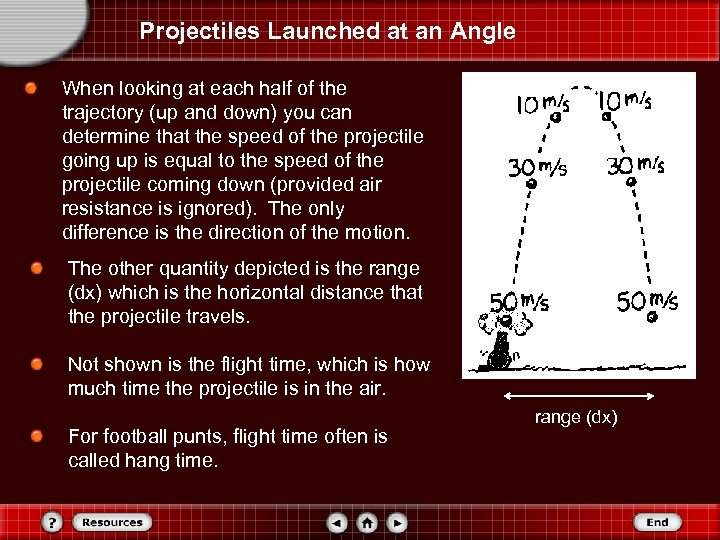Projectiles Launched at an Angle When looking at each half of the trajectory (up and down) you can determine that the speed of the projectile going up is equal to the speed of the projectile coming down (provided air resistance is ignored). The only difference is the direction of the motion. The other quantity depicted is the range (dx) which is the horizontal distance that the projectile travels. Not shown is the flight time, which is how much time the projectile is in the air. For football punts, flight time often is called hang time. range (dx)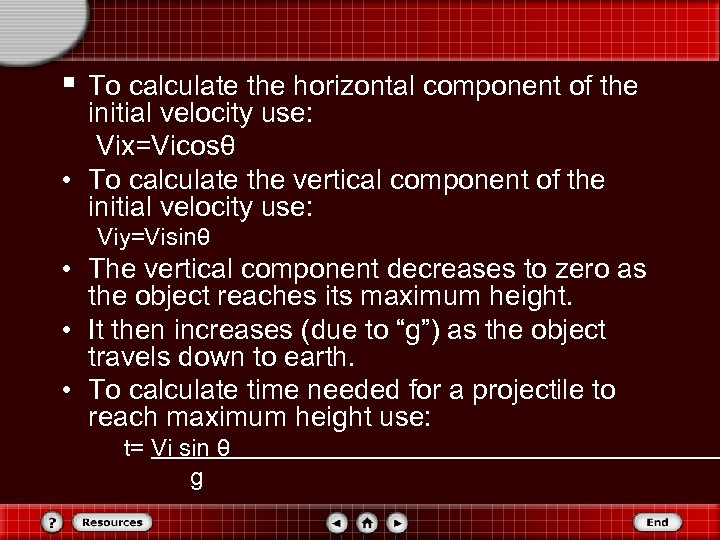§ To calculate the horizontal component of the initial velocity use: Vix=Vicosθ • To calculate the vertical component of the initial velocity use: Viy=Visinθ • The vertical component decreases to zero as the object reaches its maximum height. • It then increases (due to “g”) as the object travels down to earth. • To calculate time needed for a projectile to reach maximum height use: t= Vi sin θ g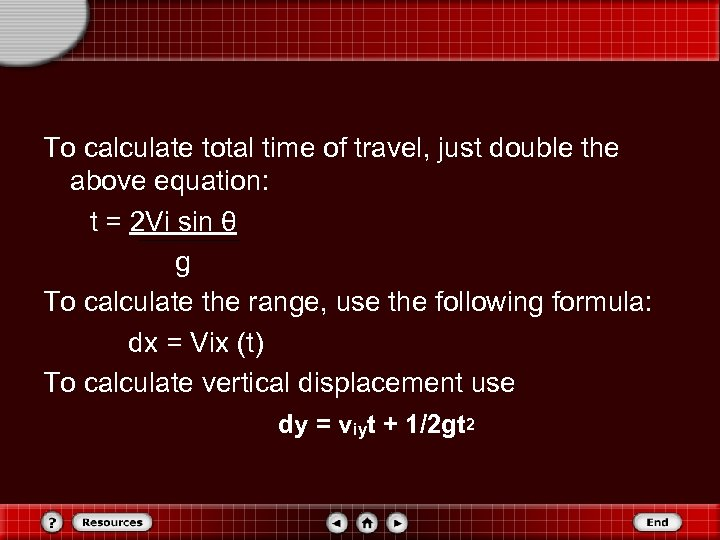To calculate total time of travel, just double the above equation: t = 2 Vi sin θ g To calculate the range, use the following formula: dx = Vix (t) To calculate vertical displacement use dy = viyt + 1/2 gt 2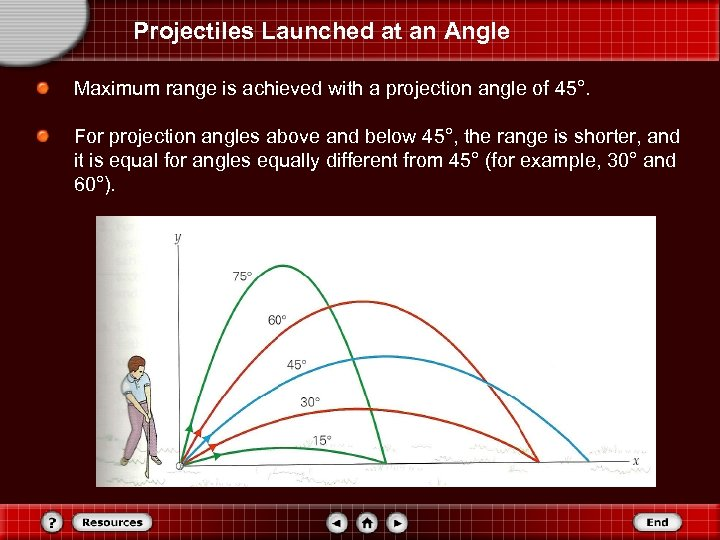Projectiles Launched at an Angle Maximum range is achieved with a projection angle of 45°. For projection angles above and below 45°, the range is shorter, and it is equal for angles equally different from 45° (for example, 30° and 60°).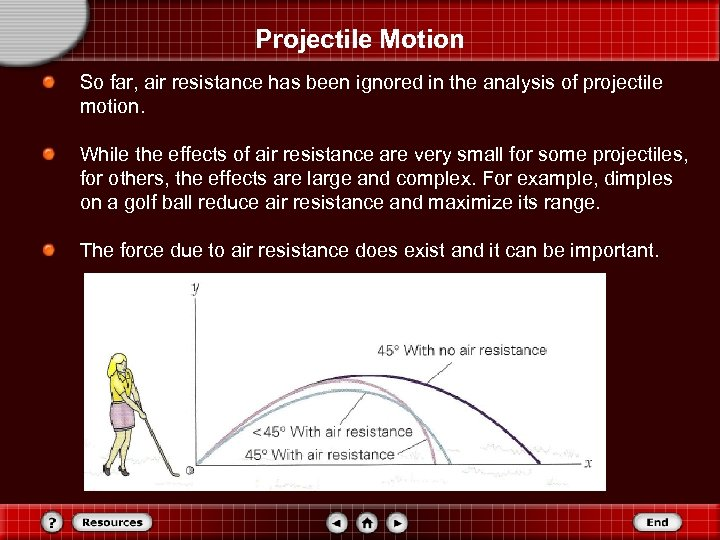Projectile Motion So far, air resistance has been ignored in the analysis of projectile motion. While the effects of air resistance are very small for some projectiles, for others, the effects are large and complex. For example, dimples on a golf ball reduce air resistance and maximize its range. The force due to air resistance does exist and it can be important.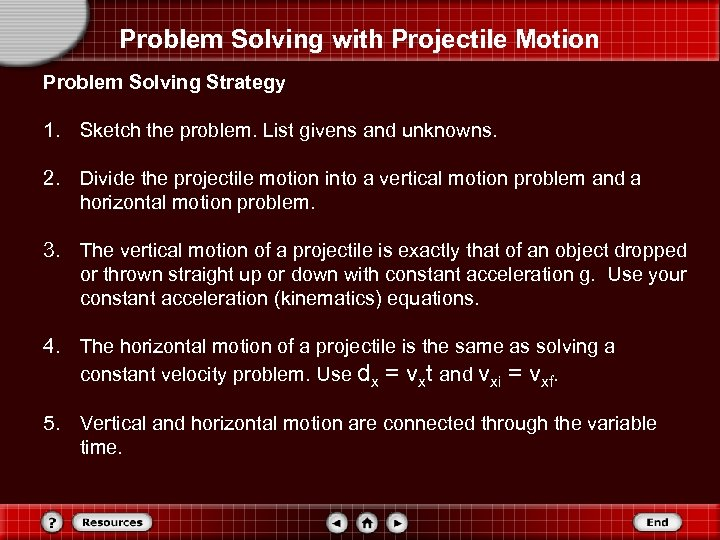Problem Solving with Projectile Motion Problem Solving Strategy 1. Sketch the problem. List givens and unknowns. 2. Divide the projectile motion into a vertical motion problem and a horizontal motion problem. 3. The vertical motion of a projectile is exactly that of an object dropped or thrown straight up or down with constant acceleration g. Use your constant acceleration (kinematics) equations. 4. The horizontal motion of a projectile is the same as solving a constant velocity problem. Use dx = vxt and vxi = vxf. 5. Vertical and horizontal motion are connected through the variable time.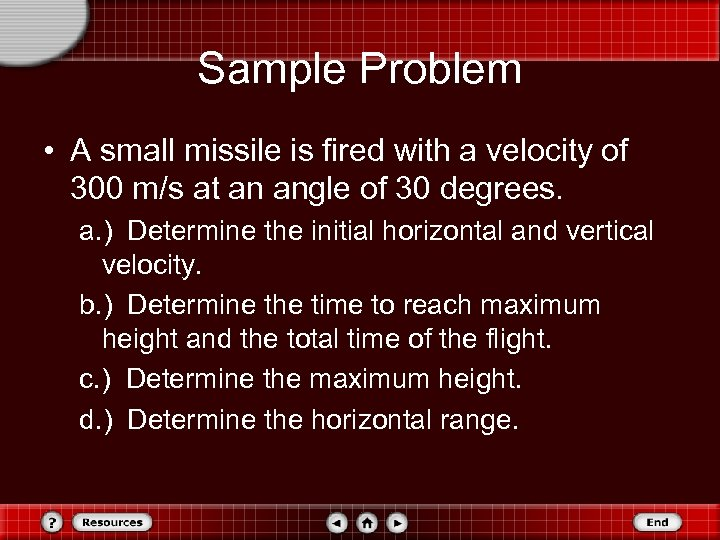Sample Problem • A small missile is fired with a velocity of 300 m/s at an angle of 30 degrees. a. ) Determine the initial horizontal and vertical velocity. b. ) Determine the time to reach maximum height and the total time of the flight. c. ) Determine the maximum height. d. ) Determine the horizontal range.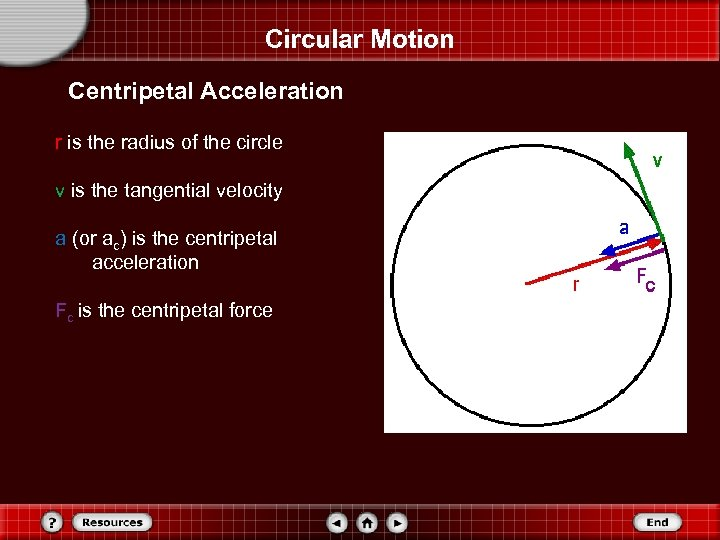Circular Motion Centripetal Acceleration r is the radius of the circle v is the tangential velocity a (or ac) is the centripetal acceleration Fc is the centripetal force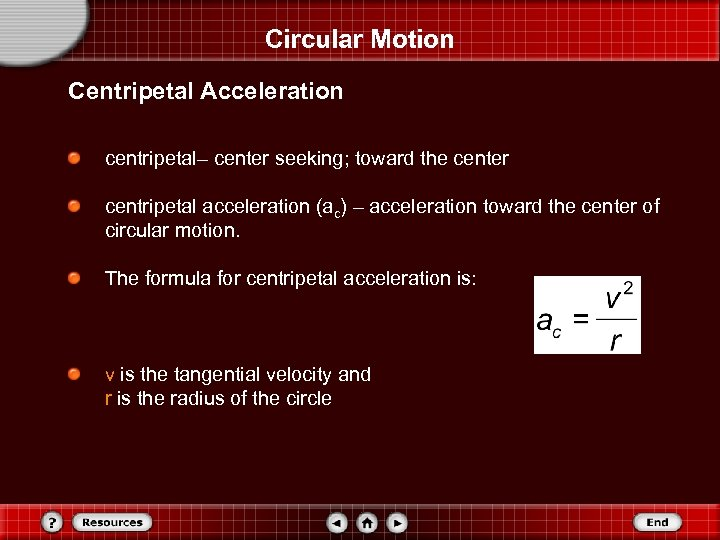Circular Motion Centripetal Acceleration centripetal– center seeking; toward the center centripetal acceleration (ac) – acceleration toward the center of circular motion. The formula for centripetal acceleration is: v is the tangential velocity and r is the radius of the circle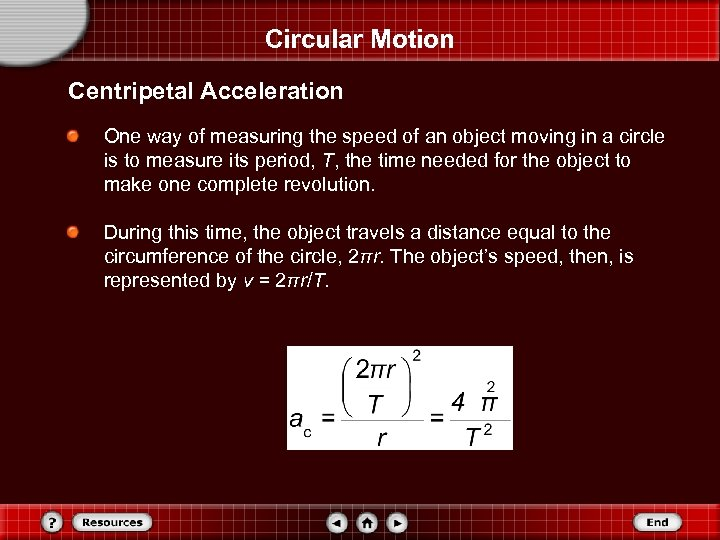Circular Motion Centripetal Acceleration One way of measuring the speed of an object moving in a circle is to measure its period, T, the time needed for the object to make one complete revolution. During this time, the object travels a distance equal to the circumference of the circle, 2πr. The object’s speed, then, is represented by v = 2πr/T.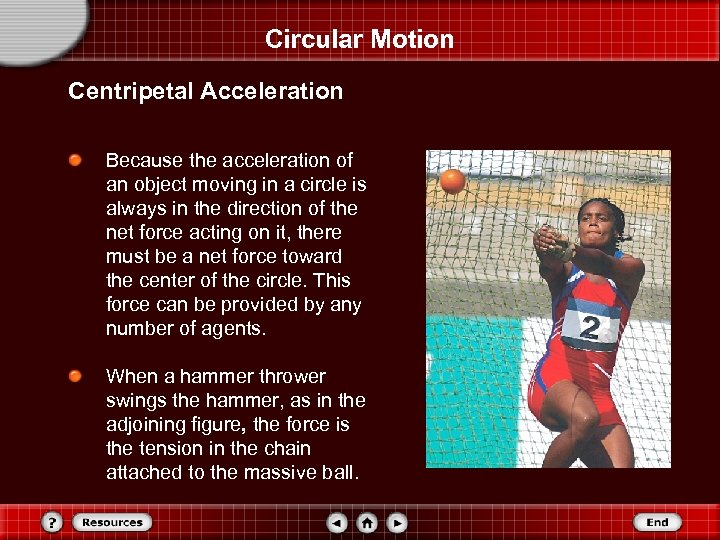Circular Motion Centripetal Acceleration Because the acceleration of an object moving in a circle is always in the direction of the net force acting on it, there must be a net force toward the center of the circle. This force can be provided by any number of agents. When a hammer thrower swings the hammer, as in the adjoining figure, the force is the tension in the chain attached to the massive ball.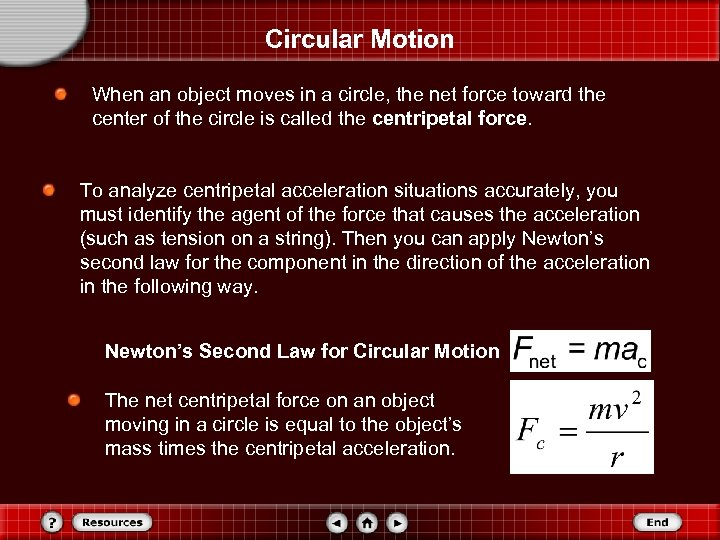Circular Motion When an object moves in a circle, the net force toward the center of the circle is called the centripetal force. To analyze centripetal acceleration situations accurately, you must identify the agent of the force that causes the acceleration (such as tension on a string). Then you can apply Newton’s second law for the component in the direction of the acceleration in the following way. Newton’s Second Law for Circular Motion The net centripetal force on an object moving in a circle is equal to the object’s mass times the centripetal acceleration.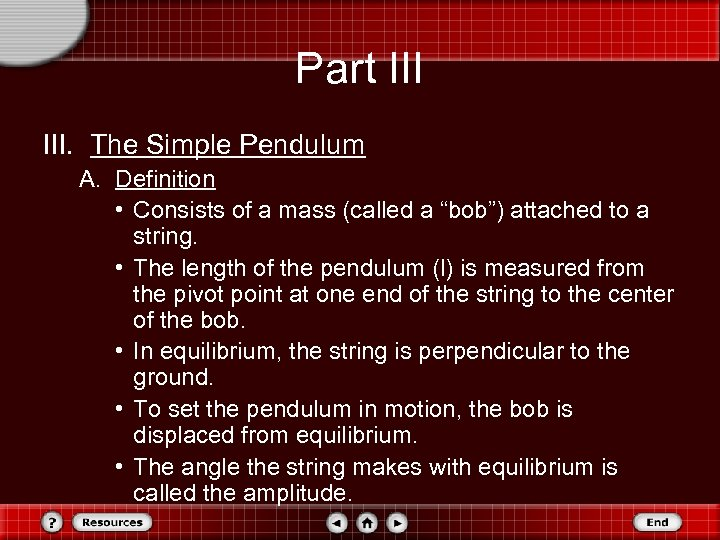Part III. The Simple Pendulum A. Definition • Consists of a mass (called a “bob”) attached to a string. • The length of the pendulum (l) is measured from the pivot point at one end of the string to the center of the bob. • In equilibrium, the string is perpendicular to the ground. • To set the pendulum in motion, the bob is displaced from equilibrium. • The angle the string makes with equilibrium is called the amplitude.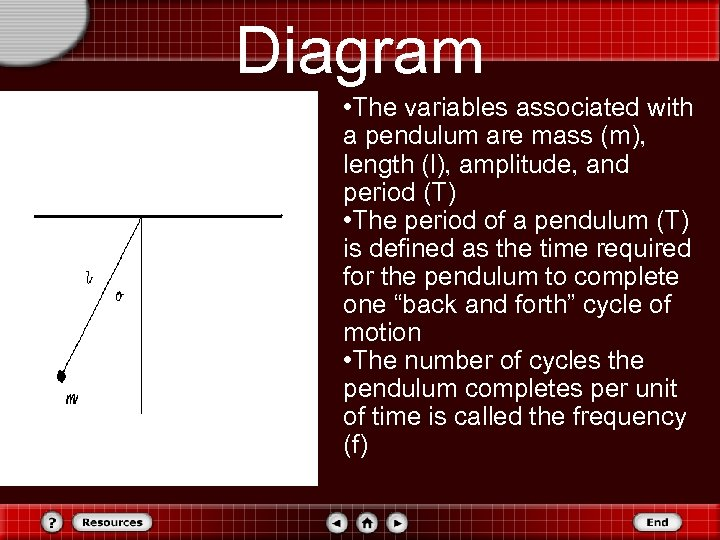Diagram • The variables associated with a pendulum are mass (m), length (l), amplitude, and period (T) • The period of a pendulum (T) is defined as the time required for the pendulum to complete one “back and forth” cycle of motion • The number of cycles the pendulum completes per unit of time is called the frequency (f)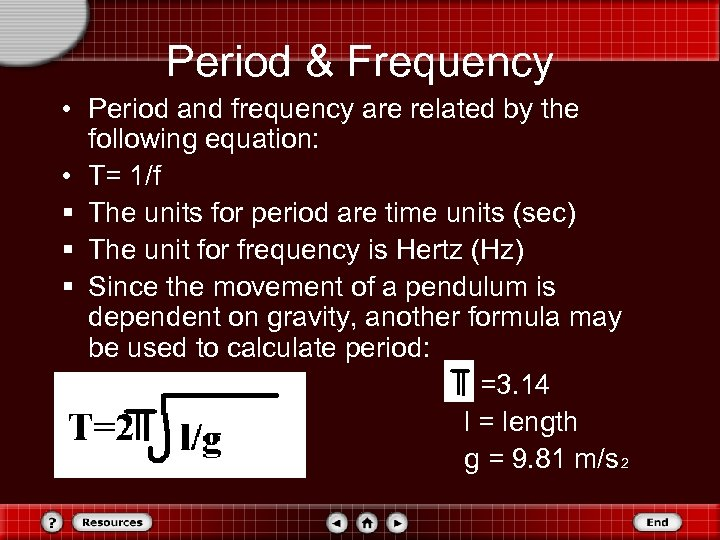Period & Frequency • Period and frequency are related by the following equation: • T= 1/f § The units for period are time units (sec) § The unit for frequency is Hertz (Hz) § Since the movement of a pendulum is dependent on gravity, another formula may be used to calculate period: =3. 14 l = length g = 9. 81 m/s 2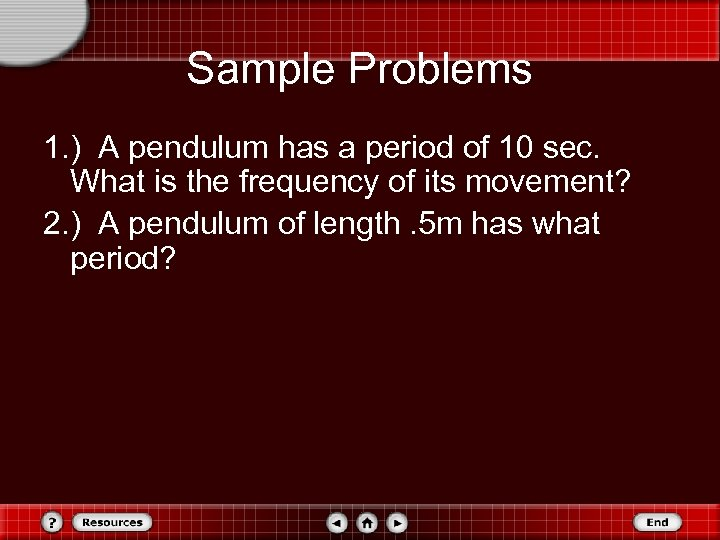Sample Problems 1. ) A pendulum has a period of 10 sec. What is the frequency of its movement? 2. ) A pendulum of length. 5 m has what period?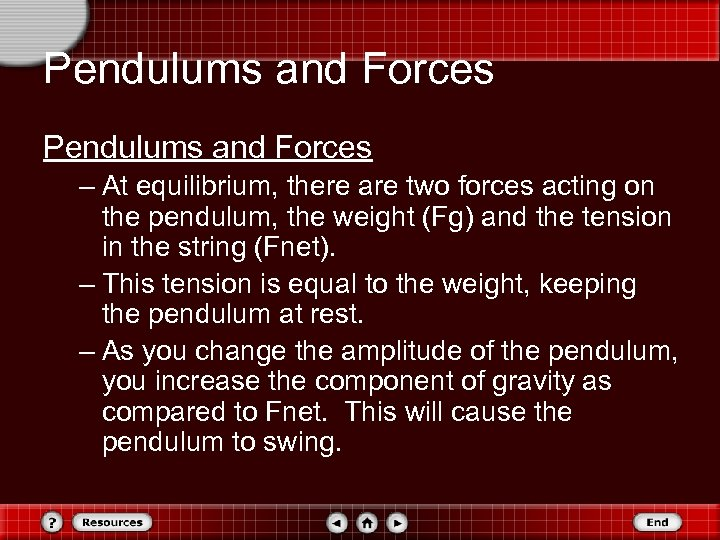Pendulums and Forces – At equilibrium, there are two forces acting on the pendulum, the weight (Fg) and the tension in the string (Fnet). – This tension is equal to the weight, keeping the pendulum at rest. – As you change the amplitude of the pendulum, you increase the component of gravity as compared to Fnet. This will cause the pendulum to swing.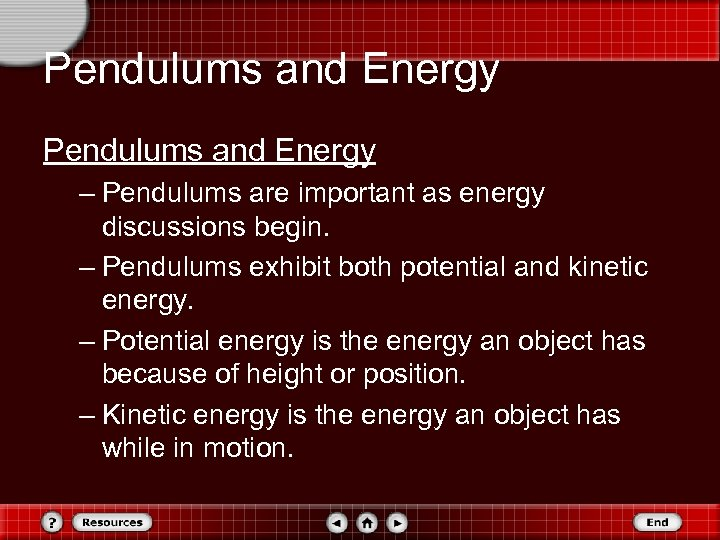Pendulums and Energy – Pendulums are important as energy discussions begin. – Pendulums exhibit both potential and kinetic energy. – Potential energy is the energy an object has because of height or position. – Kinetic energy is the energy an object has while in motion.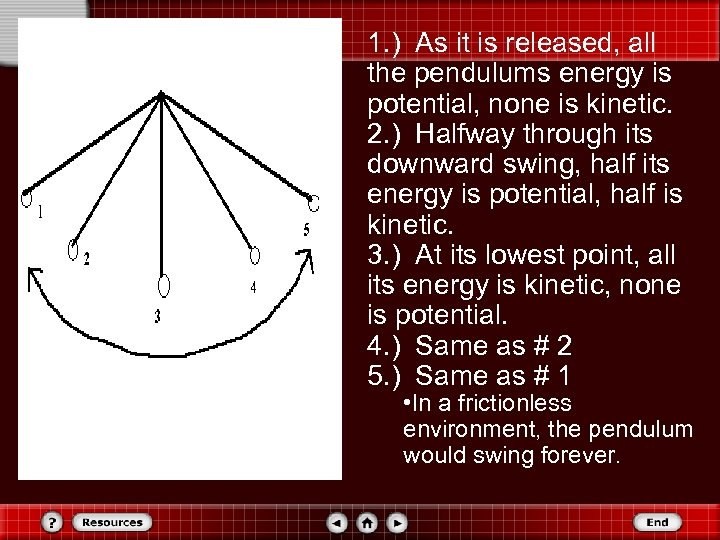1. ) As it is released, all the pendulums energy is potential, none is kinetic. 2. ) Halfway through its downward swing, half its energy is potential, half is kinetic. 3. ) At its lowest point, all its energy is kinetic, none is potential. 4. ) Same as # 2 5. ) Same as # 1 • In a frictionless environment, the pendulum would swing forever.